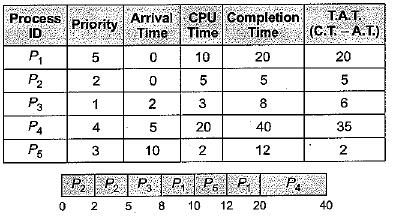Courses

# Test: Introduction, Process, Threads & CPU Scheduling- 1

## 10 Questions MCQ Test GATE Computer Science Engineering(CSE) 2022 Mock Test Series | Test: Introduction, Process, Threads & CPU Scheduling- 1

Description
This mock test of Test: Introduction, Process, Threads & CPU Scheduling- 1 for Computer Science Engineering (CSE) helps you for every Computer Science Engineering (CSE) entrance exam. This contains 10 Multiple Choice Questions for Computer Science Engineering (CSE) Test: Introduction, Process, Threads & CPU Scheduling- 1 (mcq) to study with solutions a complete question bank. The solved questions answers in this Test: Introduction, Process, Threads & CPU Scheduling- 1 quiz give you a good mix of easy questions and tough questions. Computer Science Engineering (CSE) students definitely take this Test: Introduction, Process, Threads & CPU Scheduling- 1 exercise for a better result in the exam. You can find other Test: Introduction, Process, Threads & CPU Scheduling- 1 extra questions, long questions & short questions for Computer Science Engineering (CSE) on EduRev as well by searching above.
QUESTION: 1

### Which of the following is an example of a SPOOLED device?

Solution:

Spool means simultaneous peri-pheral operations on line, a printer is a spooling device.

QUESTION: 2

### Concurrent processes are processes that

Solution:

Concurrent processes are processes that share the CPU and memory. They do overlap in time while execution. At a time CPU entertain only one process but it can switch to other without completing it as a whole.

QUESTION: 3

### Which of the following are real-time systems? 1. An on-line railway reservation system , 2. A process control system 3. Aircraft control system 4. Payroll processing system

Solution:

Real-time system are very fast and quick respondents systems. Response time of such systems is very low, to the tune of 10 ms or 100 ms or even less.

• Such systems are used where real-life scenario are being implemented like missile system, aircraft system extra.
• In process control system, the time quantum in reality is very less (to give a feel of multiprocessing so real time system is needed.
QUESTION: 4

In a time-sharing operating system, when the time slot given to a process is completed, the process goes from the RUNNING state to the

Solution: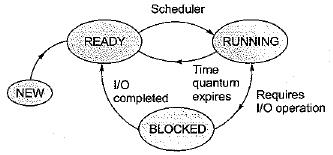In time-sharing operating system (example in Round-Robin), whenever the time slot given to a process expires, it goes back to READY state and if it requests for same I/O operation, then it goes to BLOCKED state.

QUESTION: 5

Which of the following are single-user operating system?

Solution:

MS-DOS (Microsoft Disk Operating System) is a single-user, single-tasking computer operating system that uses a command line interface. In spite of its very small size and relative simplicity, it is one of the most successful operating systems that has been developed to date.

QUESTION: 6

In a multiprogramming environment:

Solution:
• Multiprogramming environment means processor is executing multiple processes simultaneously by continuously switching between one-another.
• Therefore, multiple processes should reside in memory. However, processor can't executes more than one process at a time.
QUESTION: 7

Suppose that a process is in ‘BLOCKED’ state waiting for some I/O service. When the service is completed, it goes to the

Solution:

When process in blocked state waiting for some I/O services, whenever the services is completed it goes in the ready queue of the ready state.

QUESTION: 8

Consider a set of 5 processes whose arrival time, CPU time needed and the priority are given below: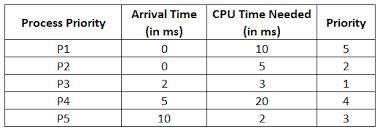Note: Smaller the number, higher the priority.

If the CPU scheduling policy is FCFS, the average waiting time will be

Solution:

According to FCFS process solve are p1 p2 p3 p4 p5 so

For p1 waiting time = 0 process time = 10 then

For p2 waiting time = (process time of p1-arrival time of p2) = 10 - 0 = 10 then

For p3 waiting time = (pr. time of (p1+p2) - arrival time of p3) = (10 + 5) - 2 = 13 and

Same for p4 waiting time = 18 - 5 = 13

Same for p5 waiting time = 38 - 10 = 28

So total average waiting time = (0 + 10 + 13 + 13 + 28) / 5

= 12.8

QUESTION: 9

Consider a set of 5 processes whose arrival time, CPU time needed and the priority are given below:Note: Smaller the number, higher the priority.

If the CPU scheduling policy is SJF, the average waiting time (without pre-emption) will be

Solution: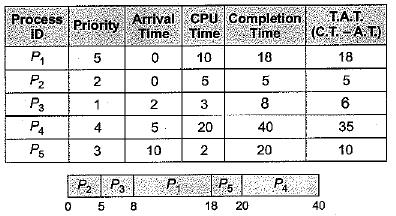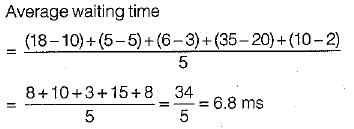QUESTION: 10

Consider a set of 5 processes whose arrival time, CPU time needed and the priority are given below:Note: Smaller the number, higher the priority.

If the CPU scheduling policy is SJF with preemption, the average waiting time will be

Solution: